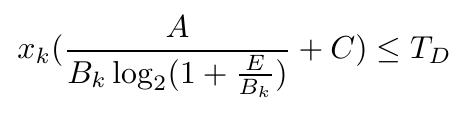# Is there anyway to represent this expression in cvx？x_k and B_k are the varaibles, 0=<x_k<=1, 0<B_k<1000. A,C,E,T_D are the constants >0. I try it in cvx, it returns :
Disciplined convex programming error:
Cannot perform the operation: {real affine} .* {convex}
How can I solve it?

No., That is non-convex. If `x_k` were fixed (and nonnegative) rather than being a variable, and `A >= 0, E >= 0`, it would be a convex constraint and could be reformulated for CVX using `inv_pos` and `rel_entr` as shown in my answer to your previous question at How to represent this expression in CVX .